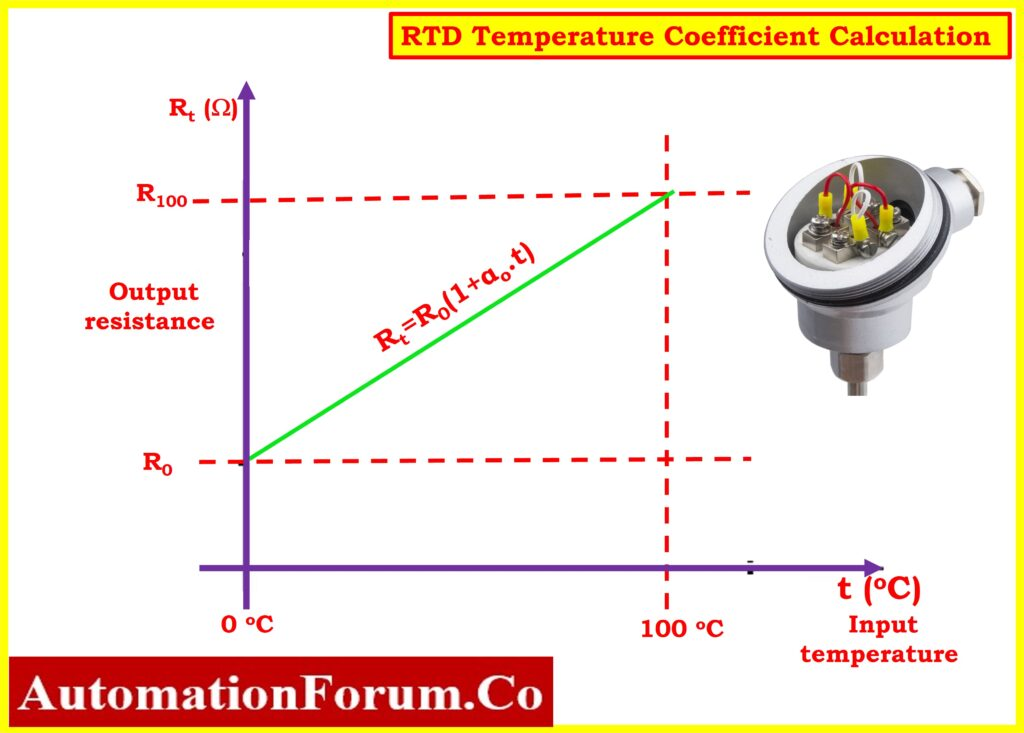# RTD Temperature Coefficient Calculation## What is the coefficient factor of RTD?

• Resistance Temperature Detectors (RTDs), also known as platinum resistance thermometers (PRTs), are widely used temperature sensors that rely on the principle that the resistance of a metal, in this case, platinum, increases with temperature.
• RTDs functions according to the theory that the resistance of a metal will increase as temperatures increase.
• The Temperature Coefficient of Resistance (TCR), denoted by αo, is a crucial parameter for RTDs.
• It represents the average resistance change per degree Celsius over a specified temperature range, usually 0°C to 100°C, divided by the resistance of the RTD, Ro, at 0°C.

## How is RTD coefficient calculated?

Here’s a step-by-step explanation of how to calculate the TCR for a PT100 RTD, along with an example calculation:

αo = (R100−R0) / (R0 X 100oC)   – Equation no. 1

R0 is the resistance of the RTD at zero degrees Celsius (ohm).

R100 is the resistance (ohm) of the RTD at 100 °C.

Note: For the purpose of this conversation, we will only be referring to RTD PT100.

The relationship between resistance (Rt) and temperature (t) for a PT100 RTD is described by the following formula:

Rt= R0(1+αo.t)   – Equation no. 2

Where:

Rt  is the RTD‘s output resistance in Ohms at temperature t.

R0 is the resistance of the RTD at 0°C (ohms).

αo is the temperature coefficient of resistance (TCR) at 0°C (per °C).

t is the temperature in degrees Celsius.

## Example Calculation

Platinum RTD PT100 has a resistance of 100 ohms at 0 degrees Celsius and 139.1 ohms at 100 degrees Celsius.

1. Find out how much resistance the RTD has at a temperature of 60 degrees Celsius.
2. Perform the TCR (Temperature Coefficient of Resistance) calculation for platinum.
3. Determine the temperature at which the resistance is 120 degrees Celsius.

## What is temperature coefficient of Pt100?

Perform the Temperature Coefficient Calculation on the RTD PT100.

From Equation no.1:

αo =  (R100−R0) / (R0 X 100oC)

αo = (139.1 -100) / (100 X 100)

αo = 0.00391 per degrees Celsius

Determine the RTD’s resistance at 60°C

From Equation no.2:

Rt= R0(1+αo.t)

R60 = Ro(1 + αt)

R60 = 100(1 + 0.00391×60)

R60 = 123.46Ω

Determine the temperature at which the resistance is 120

From Equation no.2 :

R120 = Ro(1 + αt)

120 = 100(1 + 0.00391t)

1 + 0.00391t =120/100

0.00391t = 1.2-1

t = 0.2/0.00392

t = 51.02 degrees Celsius